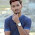## Getting Started With C++(Sumita Arora) Textbook Solutions 7th Edncredits:openclipart

Q1: What are tokens in C++? How many types of tokens allowed in C++? Exemplify your answer.

Answer: A token is the smallest unit of a c++ program that can be processed by a c++ compiler. These are also called lexical units or lexical elements. C++ has the following tokens:

• Keywords: Reserved words which have special meaning or purpose. e.g. for, const, while, class, struct etc.
• Identifiers: These are the names given to variables, constants, arrays, functions, classes or objects of a c++ program. Identifiers are case sensitive in c++. e.g. valid identifiers are: student, Date123, file234 etc.
• Literals: Data Items that never change their value during program run. Also called constants. In C++ there are five types of literals i.e. bool literal, integer constants, character constants, floating constants and string literals. e.g. 1234, 'b', 3.14 etc.
• Punctuators: These are tokens which have syntactic and semantic meaning to the compiler. e.g. {}, =, ++, -, %, *= etc.
• Operators: These are the tokens that tell the c++ compiler to perform specific mathematical or logical manipulations. C++ is full of operators. e.g. Arithmetic operators(+,-,*,/,%), Shift operators(<<. >>), Bitwise operators(&, ^, |), logical operators(&&, ||) etc.

Q2: How are keywords different from identifiers?

keywordsidentifiers
1. Keyword is a special word which carries a special meaning and purpose in c++. Keywords are reserved words.1. Identifier is the user-defined name used to identify a part of a program viz, variable, class, object, function etc. Identifiers are not reserved but programmer defined these while coding.
2. for, switch, if, else, while etc. are keywords.2. These are defined by the user but they can have letters, digits and a symbol underscore. In C++ these are case sensitive and must start with either a letter or underscore. For instance, file123, student_rec, sum etc. are identifiers in C++.
3. reserved for programming language.3. user defined but naming rules are predefined.

Q3: Identify the errors in the following code segments:
```(i) int main( )

{ cout « ”Enter two numbers”;
cin » num » auto;

(ii) #include <isostream.h>
void Main( )
{ int a,b;
cin << > ;
if (a>b) MAX=a
}

```

Answer: (i) Following errors are there in the snippet:

1. Header file <iostream.h> is missing.
2. Variables num, area, Length and breadth are not declared.
3. auto is a reserved word and it can't be used as an identifier (variable).

(ii) Following are the errors in the snippet:

1. It should be main( ) not Main( )
2. Spelling mistake in header file. It should be <iostream.h> not <isostream.h>
3. MAX has not be declared anywhere. It must be defined first before use.
4. The statement Max = a must end with punctuator semi-colon(;)
5. Statement cin << >> is invalid. The operator << must be followed by a value (variable or constant).

Q4: What are literals in C++? How many types of literals are allowed in C++?

Answer: Literals also called constants are the data items that never change their values during a program run.
C++ allows following types of literals:

1. integer literals
2. character literals
3. Floating Point constants
4. String literals
5. bool type literals

Q5: How are integer constants represented in C++? Explain with examples.

Answer: In C++, integer constants can be represented in following ways:

1. Decimal (base 10): As a decimal integer constant in which the integer constant consisting of a sequence of digits is taken to be decimal integer constant unless it begins with 0 (digit zero). For example, 1234, +98, -17 are decimal integer constants.
2. Octal Integer Constants (base 8): An octal integer constant is represented by the integer constant begins with 0. For example, decimal integer 8 will be written as 010 as octal integer.
3. Hexadecimal Integer Constant (base 16): A hexadecimal integer constant is represented by a constant which begins with 0x or 0X. For example, decimal 12 will be written as 0XB as hexadecimal integer.

Q6: What are character constants in C++? How are these implemented?

Answer: A character constant is one or more characters enclosed in single quotes, e.g. ‘p’.
There are following types of character constants:

• Single Character Constants
• Multiple Character Constants
• Escape Sequences

Single character constants e.g., ‘b’or ‘A’ have type char which is a C++ data type for characters. The value of a single character constant is the numerical value of the character in the machine’s character set. For example, the value of ‘A’ is 65 i.e. ASCII value of A.

A multi-character constant have type int, a C++  data type for integers. It is represented as enum types (not supported by old c++ compiler like Turbo C++). e.g.

enum state { waiting = 'wait', running = 'run.', stopped = 'stop' };

Escape sequences are used to display reserved character constants like newline, return key, single quotation mark etc. e.g. '\n' represents new line.

(In progress...)1.thanxx dude....

2.I need the solution for question 16

3.I need the solution for question 16

1.HAha u Dont Know this,,,

2.HAha u Dont Know this,,,

4.soooooooooooo horable

5.soooooooooooo horable

6.it help me good

7.it help me good

8.9.10.I need solution of chapter 8

11.This comment has been removed by the author.

12.13.nice but not much informations include all chapters.

14.